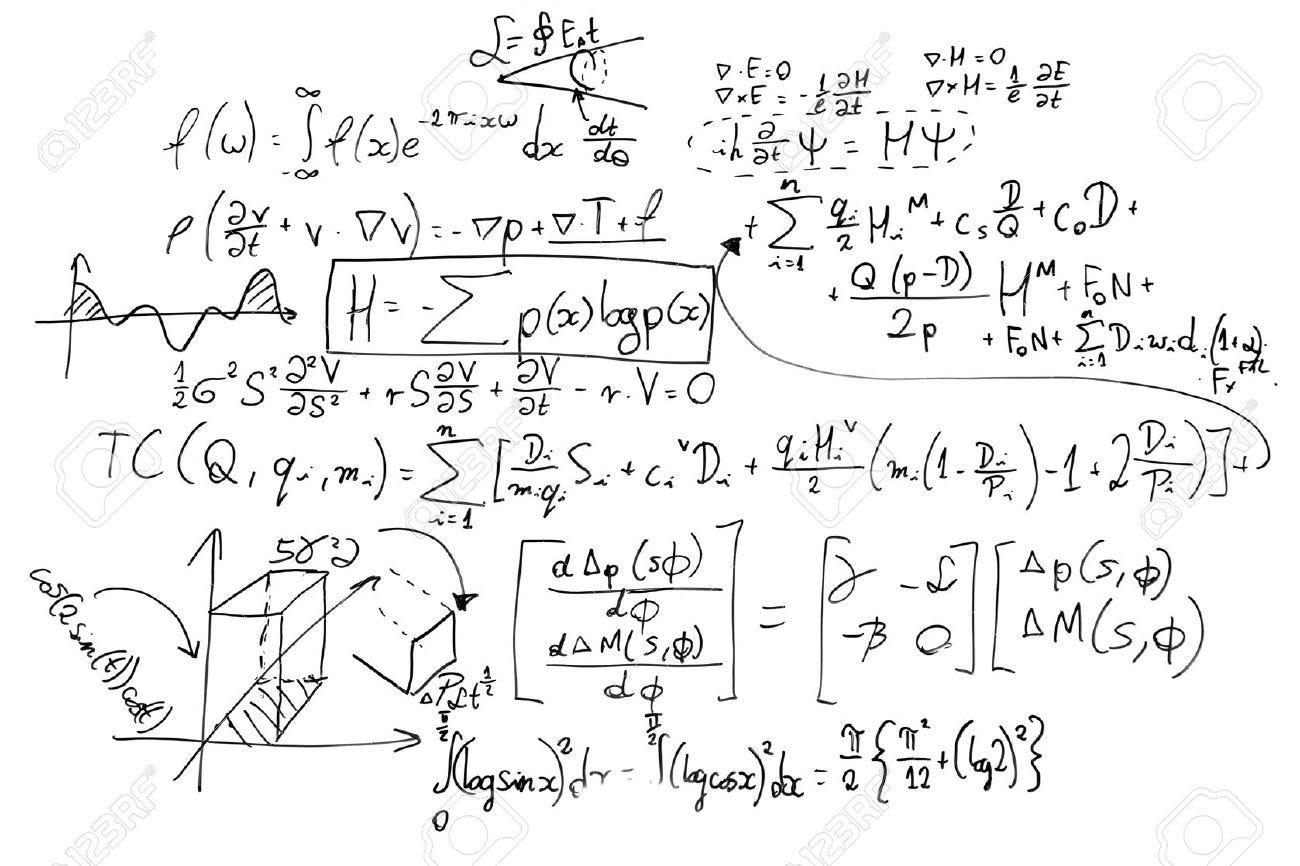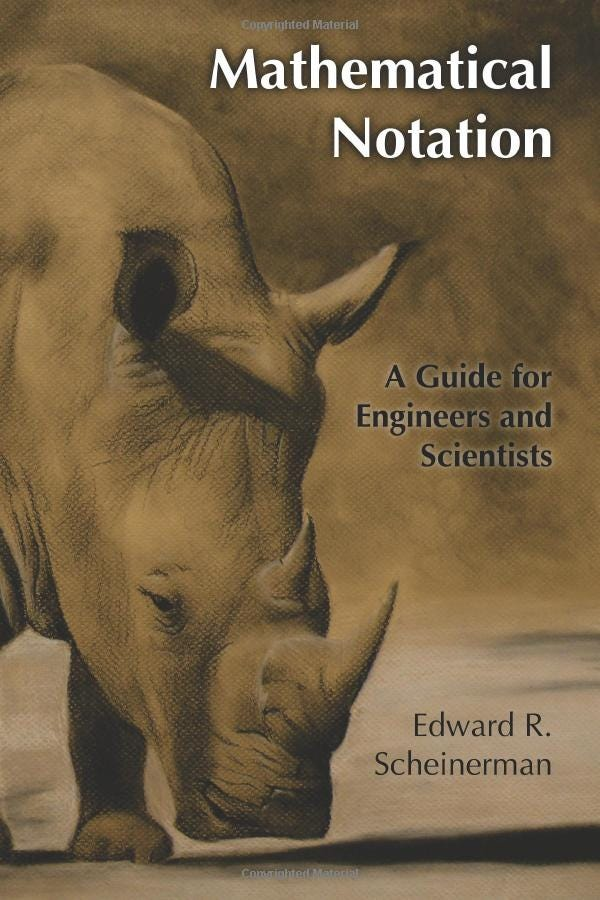# Mathematical Notation for Python Developers (Part 1)

## Mathematical Notation for Python Developers (Part 1)As a self-taught developer, I struggled most of the time reading whitepapers, or going through data science courses that contain incredible amounts of mathematical jargon (mostly weird and magic symbols).

My thanks to Edward R. Scheinerman *for the book *Mathematical Notation: A Guide for Engineers and Scientists *which was my biggest help at the start.* [A Programmer’s Guide to Creating an Eclectic Bookshelf | Data Driven Investor** Every developer should have a bookshelf. The possible set of texts in his cabinet are myriad, but not every collection…www.datadriveninvestor.com](https://www.datadriveninvestor.com/2019/03/25/a-programmers-guide-to-creating-an-eclectic-bookshelf/)

If you are struggling as I did I strongly advice you to buy it, here is a link if you feel inclined to https://www.amazon.co.uk/Mathematical-Notation-Engineers-Scientists-Scheinerman/dp/B00E321MU8.## Introduction

This tutorial is going to be focused on translating mathematical notation to Python.

NOTE: Python 3.6 is what I will be using throughout this tutorial series.

FYI: I will avoid using any libraries whenever I can so that anyone can easily understand what is going on underneath the notation.

## SETS

A set is a collection of items where repetition is forbidden.

Example: {1, 2, 3, 4}

Reinforced note: A set with repetition like {1,2,3,4,4} will be equal to {1,2,3,4} again, because repetition is non-existent within a set.

### Set declaration in Python:

``````# Declare the set
my_set = {1, 2, 3, 4, 4}
# Will output {1, 2, 3, 4}

# FUN NOTE: Strings can also be added to sets in Python like        # {"Banana", "Apple"}``````

## Set and element membership

Memberships between Sets and elements are defined with the following symbols:

For easy readability, I will add the symbol followed by it’s meaning.

∈ (element exists in), 1 ∈ {1, 2, 3}

∋ (set contains element), {1, 2, 3} ∋ 1

``````# Declare the set
my_set = {1, 2, 3}

print(1 in my_set) # Equivalent to 1 ∈ {1, 2, 3} or {1, 2, 3} ∋ 1
>> True``````

∉ (element does not exist in), 1 ∉ {2, 5, 6}

∌ (set does not contain element), {2, 5, 6} ∌ 1

``````# Declare the set
my_set = {1, 2, 3}

(1 not in my_set) # Equivalent to 1 ∉ {2, 5, 6} or {2, 5, 6} ∌ 1
>> True``````

### Dealing with large Sets

Let’s say you want to create a set that goes from 1 to 100, you do not want to write everything on paper, instead, you can show some of the elements that convey the pattern and fills the rest with ellipses (…).

Example: {1, 2, 3, 4, 5, …, 100}

You can also take the following approach:

{x ∈ ℤ: 1 ≤ x ≤ 100}

NOTES:

• ℤ = {-∞, …, -2,-1,0,1,2….∞} = the set of all integers.
• x represents a dummy variable used for each iteration.

x ∈ ℤ = x iteration is part of the ℤ set, in other words, x is gonna be an integer in each iteration.

1 ≤ x ≤ 100 = Each x iteration is going to be (greater or equal to 1) and (less or equal to 100).

``````# In case you are wondering about why the range goes to 101, that is # because, the last argument is where it stops, meaning it will only # reach the previous iteration (100).# For more information check:   # [https://www.geeksforgeeks.org/python-range-function/](https://www.geeksforgeeks.org/python-range-function/)

our_set = {x for x in range(1, 101)}
print(our_set)
>> {1, 2, 3, 4,..., 100}

# or the easiest approach for newcomers.

our_set = set()
for x in range(1, 101):
print(our_set)
>> {1, 2, 3, 4,..., 100}``````

## Set to Set Membership

### Set Equality

A = {1, 2, 3, 4, 5} and B = {1, 2, 3, 4, 5} then we can say that **A = B **because the elements found in both variables are the same.

### Subsets

A = {1, 2, 3} and B = {1, 2, 3, 4, 5}

We can see that every element in A can be found in B as well, meaning A is a subset of B or A ⊆ B = {1, 2, 3} ⊆ {1, 2, 3, 4, 5}

⊆ equivalent to“subset of”.

``````# For more info check:
# [https://www.programiz.com/python-programming/methods/set/issubset](https://www.programiz.com/python-programming/methods/set/issubset)

A = {1, 2, 3}
B = {1, 2, 3, 4, 5}
C = {1, 2, 4, 5}

# Returns True
print(A.issubset(B))

# Returns False
# B is not subset of A
print(B.issubset(A))

# Returns False
print(A.issubset(C))

# Returns True
print(C.issubset(B))``````

### Supersets

A superset is taken by the opposite order of a subset, since here we are defining that set A has **all *the elements of | is a superset of *B in which we could also say that B is a subset of A.

A ⊇ B = B ⊆ A

A = {1, 2, 3, 4, 5} and B = {1, 2, 3}

A ⊇ B = {1, 2, 3, 4, 5} ⊆ {1, 2, 3}

⊇ equivalent to “superset of”.

``````"""
[https://www.programiz.com/python-programming/methods/set/issuperset](https://www.programiz.com/python-programming/methods/set/issuperset)
"""

A = {1, 2, 3, 4, 5}
B = {1, 2, 3}
C = {1, 2, 3}

# Returns True
print(A.issuperset(B))

# Returns False
print(B.issuperset(A))

# Returns True
print(C.issuperset(B))``````